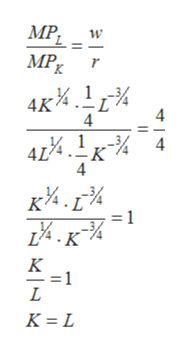# A perfectly competitive rm produces output q with capital K and labor L according to the production function: q = f(K, L) = 4K 1 4L 1 4 The price of labor is w = 4, and the price of capital is r = 4. The rm has xed costs equal to 8. The current market price is 8. (a) Prove that this production function has decreasing returns to scale. (b) Find the optimal (cost-minimizing) ratio of capital to labor inputs K L for any level of output (use scale expansion path). (c) For any q, nd the cost minimizing inputs as functions of output: i.e., derive functions L(q) and K(q).

Question
2 views

A perfectly competitive rm produces output q with capital K and labor L according to the production function: q = f(K, L) = 4K 1 4L 1 4 The price of labor is w = 4, and the price of capital is r = 4. The rm has xed costs equal to 8. The current market price is 8. (a) Prove that this production function has decreasing returns to scale. (b) Find the optimal (cost-minimizing) ratio of capital to labor inputs K L for any level of output (use scale expansion path). (c) For any q, nd the cost minimizing inputs as functions of output: i.e., derive functions L(q) and K(q).

check_circle

Step 1

Following information is given:

Step 2

a) For analyzing of it has decreasing returns to scale,

Step 3

Since, f(K , L) > f(aK , aL), the function has decreasing returns to scale. This means that, if more of input is added, the output will decrease.

b) for findi...help_outlineImage TranscriptioncloseMP _ w MP K. 4K% 4 41%. 1кИ 4 -K 4 к*.Е4 - = 1 14.к 4 K K = L fullscreen

### Want to see the full answer?

See Solution

#### Want to see this answer and more?

Solutions are written by subject experts who are available 24/7. Questions are typically answered within 1 hour.*

See Solution
*Response times may vary by subject and question.
Tagged in